# Upper And Lower Bounds Worksheet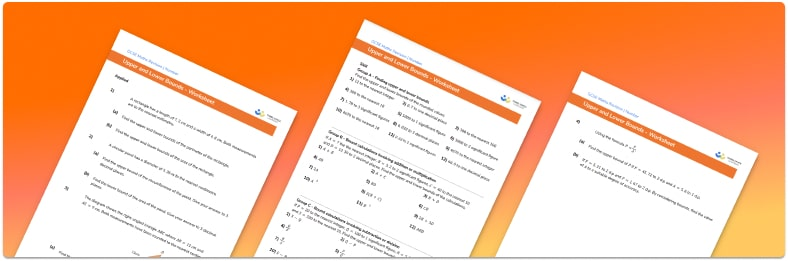Help your students prepare for their Maths GCSE with this free Upper and Lower Bounds worksheet of 44 questions and answers

• Section 1 of the Upper and Lower Bounds worksheet contains 36 skills-based Cosine Rule questions, in 3 groups to support differentiation
• Section 2 contains 4 applied Upper and Lower Bounds questions with a mix of worded problems and deeper problem solving questions
• Section 3 contains 4 foundation and higher level GCSE exam style Upper and Lower Bounds questions
• Answers and a mark scheme for all Upper and Lower Bounds questions
• Follows variation theory with plenty of opportunities for students to work independently at their own level
• All questions created by fully qualified expert secondary maths teachers
• Suitable for GCSE maths revision for AQA, OCR and Edexcel exam boards
• To receive this resource and regular emails with more free resources, blog posts and other Third Space updates, enter your email address and click below.

• This field is for validation purposes and should be left unchanged.

You can unsubscribe at any time (each email we send will contain an easy way to unsubscribe). To find out more about how we use your data, see our privacy policy.

### Upper and lower bounds at a glance

Upper & lower bounds are the maximum and minimum values a rounded number could have been before it was rounded. They are also called limits of accuracy.

When a number has been rounded, it can be useful to consider the smallest and biggest values it could have taken before it was rounded. For example, let’s say the length of a plank is given as 14cm to the nearest centimetre. Any length greater than or equal to 13.5cm would round up to 14cm. Therefore the lower bound of 14, when rounded to the nearest cm, is 13.5. Any length less than 14.5cm would round down to 14cm. For this reason we say the upper bound of 14, rounded to the nearest cm, is 14.5. The actual length of then plank could be anywhere in this range and we can represent this using an inequality: 13.5cmlength<14.5cm.

We can consider upper and lower bounds for numbers rounded to any degree of accuracy, including the nearest whole number and any number of decimal places or significant figures.

We can perform calculations involving upper and lower bounds. When calculating with upper and lower bounds we need to carefully consider how to find the overall upper or lower bound of the calculation. For example, to find an upper bound when adding, we would add two upper bounds but to find the upper bound when subtracting we would subtract a lower bound from an upper bound, giving us the biggest possible answer.

By considering upper and lower bounds we can find an answer to a calculation to a suitable degree of accuracy. The upper and lower bounds of the calculation will be the same to a given degree of accuracy so we can confidently give the final answer to that degree of accuracy.

Looking forward, students can then progress to additional number worksheets, for example aorFor more teaching and learning support on Number our GCSE maths lessons provide step by step support for all GCSE maths concepts.

## Related worksheets

Error Intervals Worksheet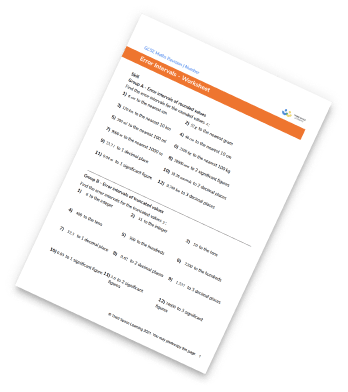Converting Mixed Numbers To Improper Fractions Worksheet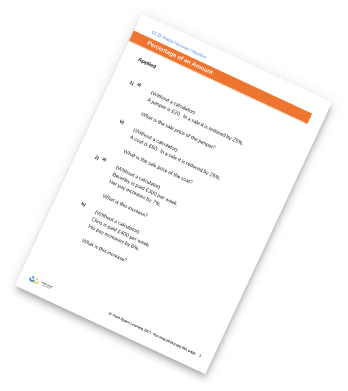Dividing Fractions Worksheet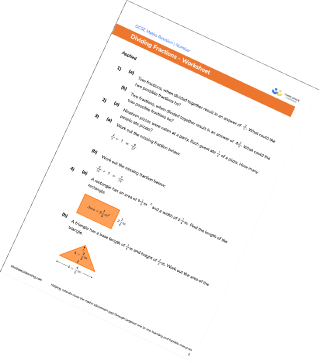Multiplying Fractions Worksheet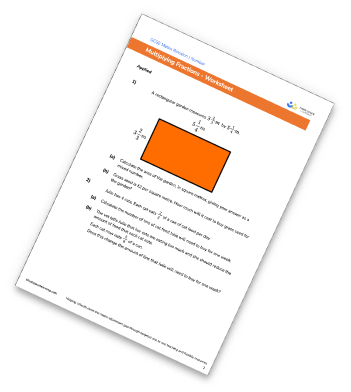## Do you have KS4 students who need more focused attention to succeed at GCSE?There will be students in your class who require individual attention to help them succeed in their maths GCSEs. In a class of 30, it’s not always easy to provide.

Help your students feel confident with exam-style questions and the strategies they’ll need to answer them correctly with our dedicated GCSE maths revision programme.

Lessons are selected to provide support where each student needs it most, and specially-trained GCSE maths tutors adapt the pitch and pace of each lesson. This ensures a personalised revision programme that raises grades and boosts confidence.

Find out more